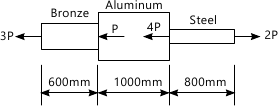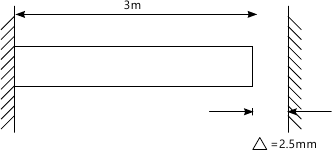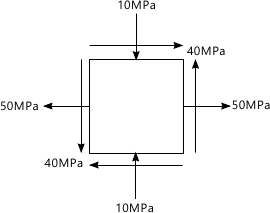MORE IN Mechanics of Materials
VTU Mechanical Engineering (Semester 3)
Mechanics of Materials
January 2012
Total marks: --
Total time: --
INSTRUCTIONS
(1) Assume appropriate data and state your reasons
(2) Marks are given to the right of every question
(3) Draw neat diagrams wherever necessary

1 (a) State the Hook's law.Neatly draw the stress-strain diagram for steel indicating all sailent points and zones on it
5 M
1 (b) A copound bar consiting of bronze.Aluminium and steel indicating loaded axially as shown in fig.Q.1(b).determine the maximum allowable value of 'P' if the change in lenghth of the bar is not to exceed 2mm and the working stresses in each material of the bar indicated in table below is not to be exceed.
 Material AreaA (mm2) Elastic modulus E(MPa) Working stress σw bronze 450 0.83×103 120 Aluminium 650 0.70×103 80 Steel 300 2 ×103 14015 M

2 (a) With standard notation ,derive an expression relate the modulus of elasticity E,Bulk Modulus K and the poission ration ?
5 M
2 (b) When a bar of 25mm diameter is subjected to a pull of 61 kN,the ,the extension on a 50mm gauge lenghth is 0.1 mm and does in diameter is 0.013mm.Calculate the value of elastic constant E,G,K and ?
8 M
2 (c) The bronze bar 3m long with 320mm2 cross sectioal area is palced between two right walls At-20 ° C there is gap ?=2.5mm as shown in fig .Q.2 (c) .Find the magnitude and the type of stress induced in the bar when it is heated to a tempreture 50.6°c.For bronze bar ,take αB = 18.0 × 10-6/°C and E=80 GPa.7 M

3 for the state of stress shown in fig.Q.3,determine :
The principle stresses and principle planes
maximum inplane sher and palne on which it is acting .Also find the normal stress on the maximum sher palne
Sketch the element aligned with plane of priciple stresses and palnes of maximum sher
also draw the Mohr's circle for the above stress satic.20 M

4 (a) Define the work and strain energy.
2 M
4 (b) A rectangular copper bar 50mm ×75mm in cross section is subjected to an axial energy of 20 Nm.Determine the minimum length of bar to limit the axial stress in the 80MPa.The modulus of elasticity of bar Is 1.15×105N/mm2
6 M
4 (c) A thin cylinder of 75mm internal diameter and 250mm long has 2.5mm thick walls. The cylinder is subjected to an internal pressure of 7MN/m2.determine the change in internal diameter and change in the length of the cylinder.Also coompter the Hoop stress and Longitudianl stress in the cylinder .Take E=2 × 105N/mm2and ?=0.3
12 M

5 (a) Derive an expression to esablish a relationship between the intensity laod to,shear force F and bending moment M in the beam
6 M
5 (b) A beam 25m long is supported at A and B and loaded as shown in fig .Q.5 Draw the sher force and bending moment diagrams for the beam computing sher force and bending moments at A,E,D,B and C find the postion and magnitude of the maximum bending moment .Also determine the point contraflexture
-----IMAGE
14 M

6 (a) State the assumption made in the simple bending theory
5 M
6 (b) A uniform I-sectio beam is 10mm wide and 150 mm deep with a flange thickness of 25mm and web thickness of 12mm the beam is simply supported over a span of 5m.it carries a uniformally distributed load intensity 83.4 kN/m throuhout its lenghth Determine the beding stress in the beam and plot the stress distribution across its cross-section
15 M

7 (a) Using the standard noation ,derive an expression for deflaction ,slope and maximum deflection of a simply supported beam 'L' subjected to a concentrated load W at its mid span
10 M
7 (b) A simply supported beam of span 20m carries two concentrated load 4 kN at 8m and 10 kN at 12m from the left end support calculate
The deflaction.
take E=2×105N/mm2 and I=109mm4
10 M

8 (a) A hollow steel shaft transmit 200Kw of power at 150 rpm.The total angel of twist in a lenghth of 5m of shaft is 3 °.Find the inner an outer diameters of the shaft if the permissimble shear stress is 60MPa.take G=80GPa
10 M
8 (b) A circular compression member is of 25mm diameter and 950mm long .Calculate the maximum buckling load.What will be the value of allowness laod if a factor of safety of 3 is expected ?Take for material of the column ?y=441MPa,E =2.07×105N/mm2
10 M

More question papers from Mechanics of Materials